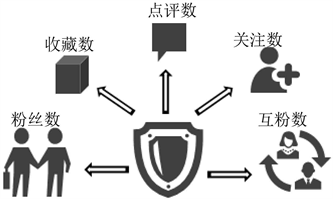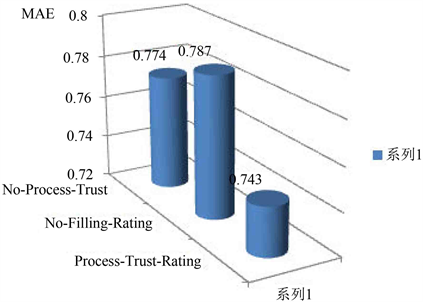# 一种基于社会化信任的改进的协同过滤推荐模型和方法 An Improved Collaborative Filtering Recommendation Model and Method Based on Social Trust

• 全文下载: PDF(1069KB)    PP.63-73   DOI: 10.12677/ECL.2018.82008
• 下载量: 198  浏览量: 315   国家自然科学基金支持

Trust relationship in the social business environment has a profound impact on consumers' purchase behavior and decision-making, and has become an important factor to support the development of online business activities. Collaborative filtering recommendation algorithm based on user history evaluation data usually faces the problem of data sparseness; that is, the sparse rating data leads to the decline of recommendation quality. In order to solve this problem, the combination of auxiliary data has become an inevitable trend. Therefore, with the development of social media, trust-based social recommendation algorithm has been proved to be an effective solution. However, most of the current algorithms directly use the binary trust relationship of the social network to improve the recommendation quality, without considering the difference in the trust strength of the user for each friend. In order to improve the accuracy of social recommendation algorithm, this paper calculates personal reliability and mutual identify reliability based on social data, and quantifies the social attention matrix based on mutual identify reliability and alleviates the data sparsity problem based on the idea of score matrix pre-filling. The experiment and analysis results based on the real data set of public comments show that the new collaborative filtering recommendation model and algorithm proposed in this paper further improve the recommendation accuracy.

1. 引言

2. 研究现状

2.1. 国外研究现状

Massa等  首次提出了基于信任的推荐系统框架，他通过将项目评分矩阵和信任关系矩阵作为系统算法的输入项并提供给评分预测模型，最终得到了预测评分矩阵，改善了传统算法数据稀疏性问题，但其采用的是未加处理的布尔型信任标注值，表达信任的能力较弱，而且由此得到的信任关系也难以抵御恶意用户的攻击。Golbeck提出了一种TidialTrust算法，该算法通过广度优先搜索(BFS)的方法对信任传播方式进行了挖掘  。Massa等  在后来对TidialTrust进一步改进，提出了一种设定最大信任传递距离的MoleTrust算法。Zarghami等  提出了一种衡量信任程度的T指数，同时引入了信任传递机制，提高了推荐的精度和覆盖率。Jamali等  将基于项目的协同过滤推荐方法与信任推荐方法相结合，提出了TrustWalker随机游走算法，该算法认为信任程度较高的邻居用户比信任程度低的邻居用户在与源用户相似的目标项目上的评分更可靠，由此对源用户的未评分目标项目进行预测评分。Chen等  在传统协同过滤算法的基础上引入了信任的传播机制，提出了TPCF信任推荐模型。Bedi等  提出了一种信任动态更新的协同过滤推荐算法，通过用户的评分相似度和共同评分数占比综合推出。

2.2. 国内研究现状

3. 基于可信评分的矩阵预处理

3.1. 可信评分计算Figure 1. The impact factor of user credibility score

1) 个人可信度评分的计算

① 点评数可信度得分

$\text{PR}_\text{CT}\left(u\right)=\frac{mf\left(u\right)}{fs\left(u\right)}×\left(\left(1-{\epsilon }_{1}\right)+{\epsilon }_{1}×{\sum }_{\begin{array}{l}v=1\\ v\ne u\end{array}}^{k}\mathrm{lg}\frac{ct\left(v\right)}{|ct\left(v\right)-ct\left(u\right)|}\right)$ (1)

② 粉丝数可信度得分

$\text{PR}_\text{FS}\left(u\right)=\frac{mf\left(u\right)}{fs\left(u\right)}×\left(\left(1-{\epsilon }_{2}\right)+{\epsilon }_{2}×{\sum }_{\begin{array}{l}v=1\\ v\ne u\end{array}}^{k}\mathrm{lg}\frac{fs\left(v\right)}{|fs\left(v\right)-fs\left(u\right)|}\right)$ (2)

③ 关注数可信度得分

$\text{PR}_\text{FW}\left(u\right)=\frac{mf\left(u\right)}{fs\left(u\right)}×\left(\left(1-{\epsilon }_{3}\right)+{\epsilon }_{3}×{\sum }_{\begin{array}{l}v=1\\ v\ne u\end{array}}^{k}\mathrm{lg}\frac{fw\left(v\right)}{|fw\left(v\right)-fw\left(u\right)|}\right)$ (3)

④ 收藏数可信度得分

$\text{PR}_\text{CN}\left(u\right)=\frac{mf\left(u\right)}{fs\left(u\right)}×\left(\left(1-{\epsilon }_{1}\right)+{\epsilon }_{4}×{\sum }_{\begin{array}{l}v=1\\ v\ne u\end{array}}^{k}\mathrm{lg}\frac{cn\left(v\right)}{|cn\left(v\right)-cn\left(u\right)|}\right)$ (4)

⑤ 互粉数可信度得分

$\text{PR}_\text{MF}\left(u\right)=\frac{mf\left(u\right)}{fs\left(u\right)}×\left(\left(1-{\epsilon }_{5}\right)+{\epsilon }_{5}×{\sum }_{\begin{array}{l}v=1\\ v\ne u\end{array}}^{k}\mathrm{lg}\frac{mf\left(v\right)}{|mf\left(v\right)-mf\left(u\right)|}\right)$ (5)

$\begin{array}{l}{\epsilon }_{1}=\frac{ct\left(v\right)}{\mathrm{max}\left[ct\left(n\right)\right]},{\epsilon }_{2}=\frac{fs\left(v\right)}{\mathrm{max}\left[fs\left(n\right)\right]},{\epsilon }_{3}=\frac{fw\left(v\right)}{\mathrm{max}\left[fw\left(n\right)\right]},\\ {\epsilon }_{4}=\frac{cn\left(v\right)}{\mathrm{max}\left[cn\left(n\right)\right]},{\epsilon }_{5}=\frac{mf\left(v\right)}{\mathrm{max}\left[mf\left(n\right)\right]},n=1,2,\cdot \cdot \cdot ,k\end{array}$ (6)

$\text{PR}\left(u\right)=\text{PR}_\text{CT}\left(u\right)+\text{PR}_\text{FS}\left(u\right)+\text{PR}_\text{FW}\left(u\right)+\text{PR}_\text{CN}\left(u\right)+\text{PR}_\text{MF}\left(u\right)$ (7)

2)互鉴可信度评分的计算

$\text{MI}\left(u\right)=1-\rho +\rho ×{\sum }_{\begin{array}{l}v=1\\ v\ne u\end{array}}^{k}\mathrm{lg}\frac{\text{PR}\left(v\right)}{|\text{PR}\left(v\right)-\text{PR}\left(u\right)|},u=1,2,\cdot \cdot \cdot ,k$ (8)

3.2. 社交关注矩阵可信量化Table 1. A social attention matrix based on mutual trust

3.3. 项目评分矩阵预填充

$\text{FR}\left(u,v\right)=\frac{|{\text{UI}}_{u,v}|}{|{\text{PI}}_{u,v}|}$ (9)

${P}_{u,i}=\stackrel{¯}{{r}_{u}}+\text{MI}\left(v\right)×\left({r}_{v,i}-\stackrel{¯}{{r}_{v}}\right)$ (10)

${{r}^{\prime }}_{u,i}=\stackrel{¯}{{r}_{u}}+\frac{{\sum }_{k\in RNu}\text{FR}\left(u,k\right)×\left({r}_{k,i}-\stackrel{¯}{{r}_{k}}\right)}{{\sum }_{k\in RNu}\text{FR}\left(u,k\right)}$ (11)

4. 基于可信评分预估矩阵的相似性计算

4.1. 用户相似性计算

$\text{SIM}_\text{A}\left(u,v\right)=\frac{{\sum }_{k\in {\text{PI}}_{u,v}}\left({r}_{u,k}-\stackrel{¯}{{r}_{u}}\right)\cdot \left({r}_{v,k}-\stackrel{¯}{{r}_{v}}\right)}{\sqrt{{\sum }_{k\in {\text{PI}}_{u,v}}\left({r}_{u,k}-\stackrel{¯}{{r}_{u}}\right)}\cdot \sqrt{{\sum }_{k\in {\text{PI}}_{u,v}}\left({r}_{v,k}-\stackrel{¯}{{r}_{v}}\right)}}$ (12)

$\text{Sim}_\text{A}\left(u,v\right)=\frac{1+\text{Sim}_\text{A}\left(u,v\right)}{2}$ (13)

4.2. 预测评分计算

${P}_{u,i}=\stackrel{¯}{{r}_{u}}+\frac{{\sum }_{v\in \text{NS}\left(u\right),\text{RS}\left(i\right)}\text{Sim}_\text{A}\left(u,v\right)×\left({r}_{v,i}-\stackrel{¯}{{r}_{v}}\right)}{{\sum }_{v\in \text{NS}\left(u\right),\text{RS}\left(i\right)}\text{Sim}_\text{A}\left(u,v\right)}$ (14)

5. 算法实施步骤Figure 2. The implementation step diagram of the algorithm in this paper

STEP1-1可信评分的计算：根据推荐系统的用户数据库中，提取用户的点评数，关注数，粉丝数，收藏数，利用公式(1)至公式(7)离线计算每一位用户的五种影响因子得分和个人可信度评分，再利用公式(8)计算出每一位用户的互鉴可信度评分。

STEP1-2社交关注矩阵可信量化：将STEP1-1中计算出来的互鉴可信度融入到初始社交关注关系矩阵中，如表1所示，得到改善可信水平后的社会化信任矩阵。

STEP1-3项目评分矩阵预填充：将STEP1-1输出的互鉴可信度利用公式(9)至公式(11)，先转化为预估可信度后对用户未评分项目进行评分预测，并补充到初始用户项目评分矩阵的相应位置，最后得到数据密度改善的用户项目评分矩阵。

STEP2基于信任传播的相似性计算：基于STEP1-3填充后的项目评分矩阵，利用公式(12)至公式(13)计算相似度矩阵。

STEP3预测评分计算：根据公式(14)对用户的评分进行预测，将预测评分按照降序排序后形成推荐列表，位于前列的是目标用户最可能乐意接受的项目，以此进行实时的在线推荐。

6. 实验及结果分析

6.1. 实验数据集和评估标准Table 2. Characteristic statistics of experimental data set

6.2. 实验结果讨论

1) 可信评分影响因子充分合理性验证

2) 社交关注和评分矩阵处理影响验证Table 3. Calculating MAE results with different influence factors of credible score

MAE值取五个训练数据分组集计算的平均结果。测试分为三种情况：

① 未可信量化社交关注矩阵但预填充评分矩阵(No-Process-Trust)；

② 未预填充评分矩阵但可信量化社交关注矩阵(No-Filling-Rating)；

③ 既量化社交关注矩阵又预填充评分矩阵(Process-Trust-Rating)。Figure 3. The impact of social attention and score matrix processing on MAE

7. 结论及展望

  Ma, H., Yang, H.-X. and Michael, R. (2008) SoRec: Social Recommendation Using Probabilistic Matrix Factorization. In: Proceedings of the 17th ACM Conference on Information and Knowledge Management, IEEE Press, New York, 931-941. https://doi.org/10.1145/1458082.1458205  冯勇, 李军平, 徐红艳, 等. 基于社会网络分析的协同推荐方法改进[J]. 计算机应用, 2013, 33(3): 841-844.  Massa, P. and Avesani, P. (2007) Trust-Aware Rec-ommender Systems. Proceedings of the 2007 ACM Conference on Recommender Systems, Minneapolis, 19-20 October 2007, 17-24. https://doi.org/10.1145/1297231.1297235  俞琰, 邱广华. 用户兴趣变化感知的重启动随机游走推荐算法研究[J]. 现代图书情报技术, 2012(4): 48-53.  Mohsen, J. and Martin, E. (2010) A Matrix Factor-ization Technique with Trust Propagation for Recommendation in Social Networks. Proceedings of the Fourth ACM Conference on Recommender Systems, Barcelona, 26-30 September 2010, 135-142.  Yang, X.-W., Steck, H. and Liu, Y. (2012) Circle-Based Recommendation in Online Social Networks. Proceedings of the 18th ACM SIGKDD In-ternational Conference on Knowledge Discovery and Data Mining, Beijing, 12-16 August 2012, 155-165. https://doi.org/10.1145/2339530.2339728  Ma, H., Zhou, D.-Y. and Liu, C. (2011) Recommender Systems with Social Regularization. Proceedings of the 4th ACM International Conference on Web Search and Data Mining, Hong Kong, 9-12 February 2011, 287-297. https://doi.org/10.1145/1935826.1935877  李春, 朱珍敏, 高晓芳. 基于邻居决策的协同过滤推荐算法[J]. 计算机工程, 2010, 36(13): 34-36.  罗辛, 欧阳元新, 熊璋. 通过相似度支持度优化基于K近邻的协同过滤算法[J]. 计算机学报, 2010, 33(8): 1437-1445.  Massa, P. and Bhatacharjee, B. (2005) Using Trust in Recommender Systems: An Experimental Analysis. Proceedings of Conference on Trust Management, Paris, 23-26 May 2005, 221-235. https://doi.org/10.1007/978-3-540-24747-0_17  Golbeck, J.A. (2005) Computing and Applying Trust in Web-Based Social Networks. Ph.D. Thesis.  Zarghami, A., Fazeli, S., Dokoohaki, N., et al. (2009) Social Trust-Aware Recommendation System: A T-Index Approach. Proceedings of the 2009 IEEE/WIC/ACM International Joint Conference on Web Intelligence and Intelligent Agent Technologies, Milan, 15-18 September 2009, 85-90. https://doi.org/10.1109/WI-IAT.2009.237  Jamali, M. and Ester, M. (2009) Trustwalker: A Random Walk Model for Combining Trust-Based and Item-Based Recommendation. Proceedings of the 15th ACM SIGKDD Interna-tional Conference on Knowledge Discovery and Data Mining, Paris, 28 June-1July 2009, 397-406. https://doi.org/10.1145/1557019.1557067  Chen, X.C., Liu, R.J. and Chang, H.Y. (2010) Research of Col-laborative Filtering Recommendation Algorithm Based on Trust Propagation Model. Computer Application and System Modeling, Taiyuan, 22-24 October 2010, Vol. 4,177-183.  Bedi, P. and Sharma, R. (2012) Trust Based Recom-mender System Using Ant Colony for Trust Computation. Expert Systems with Applications, 39, 1183-1190. https://doi.org/10.1016/j.eswa.2011.07.124  唐文, 陈钟. 基于模糊集合理论的主观信任管理模型研究[J]. 软件学报, 2003(8): 1401-1408.  陈婷, 朱青, 周梦溪, 等. 社交网络环境下基于信任的推荐算法[J]. 软件学报, 2017, 28(3): 721-731.  潘一腾, 何发智, 于海平. 一种基于信任关系隐含相似度的社会化推荐算法[J]. 计算机学报, 2018, 41(1): 65-81.  王瑞琴, 蒋云良, 李一啸, 等. 一种基于多元社交信任的协同过滤推荐算法[J]. 计算机研究与发展, 2016, 53(6): 1389-1399.  王占, 林岩. 基于信任与用户兴趣变化的协同过滤方法研究[J]. 情报学报, 2017, 36(2): 197-205.  吴应良, 姚怀栋, 李成安. 一种引入间接信任关系的改进协同过滤推荐算法[J]. 现代图书情报技术, 2015(9): 38-45.  杜巍, 高长元. 基于个性化情景的移动商务信任推荐模型研究[J]. 情报科学, 2017, 35(10): 23-29.  陈梅梅, 薛康杰. 基于标签簇多构面信任关系的个性化推荐算法研究[J]. 数据分析与知识发现, 2017, 1(5): 94-101.  薛福亮, 刘君玲. 基于用户间信任关系改进的协同过滤推荐方法[J]. 数据分析与知识发现, 2017, 1(7): 90-99.  闫光辉, 刘晓飞, 王梦阳. 基于链接的微博用户可信度研究[J]. 计算机应用研究, 2015, 32(10): 2910-2913+2917.